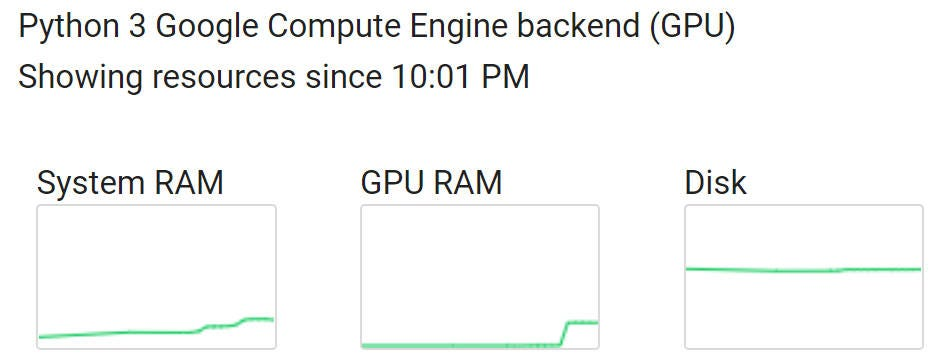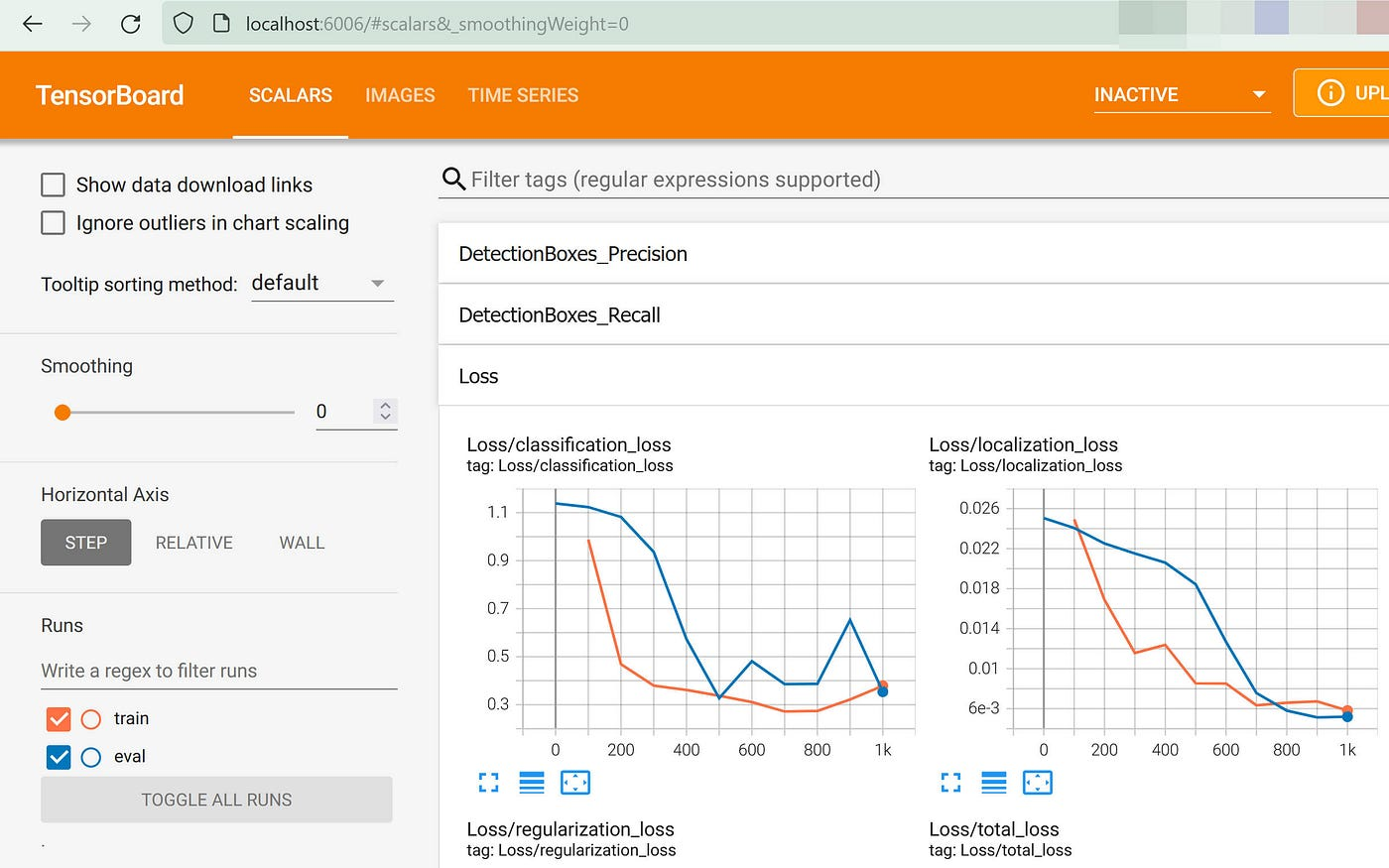# Make Tensorflow’s Object-Detection Validation a true Post-Process

`1). By default, how is Training and Validation supposed to intertwine?2). The Pros & Cons of the aforementioned approach3). Make Validation a true Post-process4). Verify5). Conclusion`

# By default, how is Training and Validation supposed to intertwine?

`python model_main_tf2.py \  --pipeline_config_path="some_path/pipeline.config" \  --model_dir="some_path" \  --checkpoint_every_n=100 \  --num_train_steps=1000 \  --alsologtostderr`
`python model_main_tf2.py \  --pipeline_config_path="some_path/pipeline.config" \  --model_dir="some_path" \  --checkpoint_dir="some_path" \  --eval_timeout=60`

# The Pros & Cons of the aforementioned approach

`# 1). module "model_main_tf2.py", line 81.if FLAGS.checkpoint_dir: # if checkpoint_dir argument is used.  model_lib_v2.eval_continuously(...# 2). module "model_lib_v2.py", function eval_continuously(), line 1136:for latest_checkpoint in tf.train.checkpoints_iterator(  checkpoint_dir, timeout=timeout, min_interval_secs=wait_interval):# 3). module "checkpoint_utils.py", function checkpoints_iterator(), line 194:while True:  new_checkpoint_path = wait_for_new_checkpoint(    checkpoint_dir, checkpoint_path, timeout=timeout)# 4). same module, function wait_for_new_checkpoint(), line 139:while True:  checkpoint_path = checkpoint_management.latest_checkpoint(checkpoint_dir)`
• Pros:
- No need to keep all Checkpoints (In my case, one Checkpoint costs 42.5 MB). Indeed, Training only maintains the last 7 Checkpoints. This configuration is not changeable from outside.
- Combining with Tensorboard in real-time, one can stop the Training early if e.g. `loss` is no longer substantially reduced.
• Cons:
- Resources must be shared between Training and Validation, which can slow down the Training. The Validation could also use GPU (Picture ).
- Validation process only takes into account the latest Checkpoint. In some cases, e.g. large Validation set and more granular `checkpoint_every_n`, the Validation may miss some Checkpoints, resulting in less fine-grained Visualization for Validation data.
- Complexity: In CoLab, it’s not straightforward to run multiple cells in parallel (i.e. one cell for Training, one cell for Validation).Picture 1: GPU is used during Validation

# Make Validation a true Post-process

`# 1). module "model_main_tf2.py", line 105:with strategy.scope():  model_lib_v2.train_loop(    pipeline_config_path=FLAGS.pipeline_config_path,    model_dir=FLAGS.model_dir,    train_steps=FLAGS.num_train_steps,    use_tpu=FLAGS.use_tpu,    checkpoint_every_n=FLAGS.checkpoint_every_n,    record_summaries=FLAGS.record_summaries)# 2). module "model_lib_v2.py", function train_loop(), line 443:def train_loop(  pipeline_config_path,  model_dir,  config_override=None,  train_steps=None,  use_tpu=False,  save_final_config=False,  checkpoint_every_n=1000,  checkpoint_max_to_keep=7,  record_summaries=True,  performance_summary_exporter=None,  num_steps_per_iteration=NUM_STEPS_PER_ITERATION,  **kwargs):`
`for latest_checkpoint in tf.train.checkpoints_iterator(    checkpoint_dir, timeout=timeout, min_interval_secs=wait_interval):  ...ckpt.restore(latest_checkpoint).expect_partial()`
`import regex as reimport globdef natural_sort(l):   convert = lambda text: int(text) if text.isdigit() else text.lower()   alphanum_key = lambda key: [ convert(c) for c in re.split('([0-9]+)', key) ]   return sorted(l, key = alphanum_key)for latest_checkpoint in natural_sort(list(set(map(lambda n: n[:n.index(".")], glob.glob(f"{checkpoint_dir}/ckpt-*.*"))))):`

# Verify

`tensorboard --logdir=checkpoint_dir`Picture 2: Visualization for Training & Validation

--

--